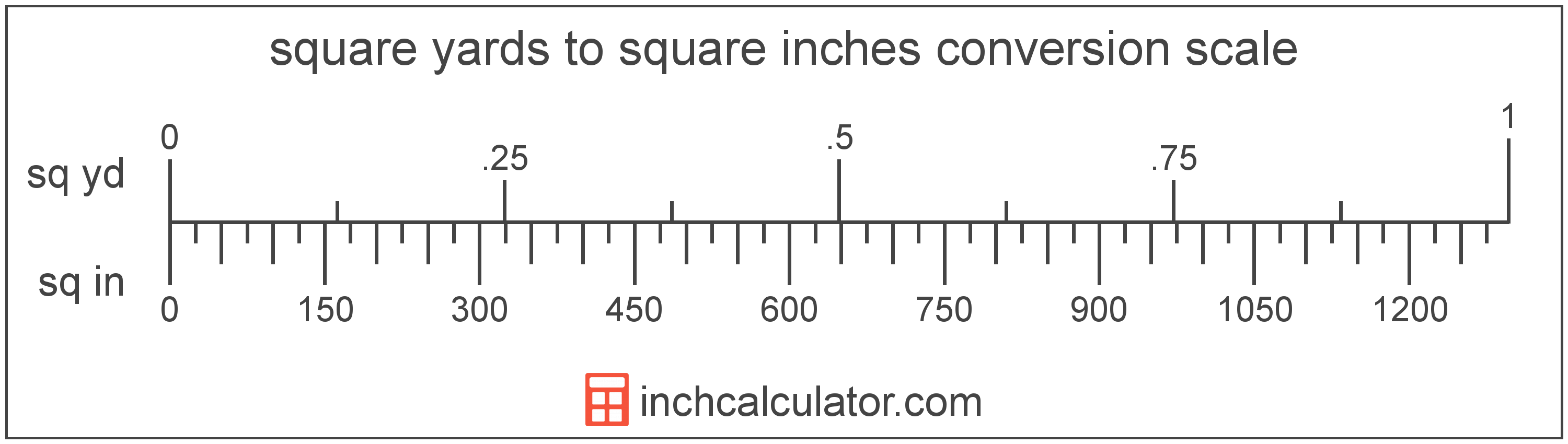# Square Yards to Square Inches Converter

Enter the area in square yards below to get the value converted to square inches.

(find square yards)
Results in Square Inches:1 sq yd = 1,296 sq in

Do you want to convert square inches to square yards?

## How to Convert Square Yards to Square Inches

To convert a measurement in square yards to a measurement in square inches, multiply the area by the following conversion ratio: 1,296 square inches/square yard.

Since one square yard is equal to 1,296 square inches, you can use this simple formula to convert:

square inches = square yards × 1,296

The area in square inches is equal to the area in square yards multiplied by 1,296.

For example, here's how to convert 5 square yards to square inches using the formula above.
square inches = (5 sq yd × 1,296) = 6,480 sq in### How Many Square Inches Are in a Square Yard?

There are 1,296 square inches in a square yard, which is why we use this value in the formula above.

1 sq yd = 1,296 sq in

## What Is a Square Yard?

One square yard is equivalent to the area of a square with sides that are each 1 yard in length. One square yard is roughly equal to 9 square feet or 0.836127 square meters.

The square yard is a US customary and imperial unit of area. A square yard is sometimes also referred to as a square yd. Square yards can be abbreviated as sq yd, and are also sometimes abbreviated as yd². For example, 1 square yard can be written as 1 sq yd or 1 yd².

You can use a square yards calculator to calculate the area of a space if you know its dimensions.

## What Is a Square Inch?

One square inch is equivalent to the area of a square with sides that are each 1 inch in length. One square inch is equal to 1/144 of a square foot or 1/1,296 of a square yard.

The square inch is a US customary and imperial unit of area. A square inch is sometimes also referred to as a square in. Square inches can be abbreviated as sq in, and are also sometimes abbreviated as in². For example, 1 square inch can be written as 1 sq in or 1 in².

## Square Yard to Square Inch Conversion Table

Table showing various square yard measurements converted to square inches.
Square Yards Square Inches
0.001 sq yd 1.296 sq in
0.002 sq yd 2.592 sq in
0.003 sq yd 3.888 sq in
0.004 sq yd 5.184 sq in
0.005 sq yd 6.48 sq in
0.006 sq yd 7.776 sq in
0.007 sq yd 9.072 sq in
0.008 sq yd 10.37 sq in
0.009 sq yd 11.66 sq in
0.01 sq yd 12.96 sq in
0.02 sq yd 25.92 sq in
0.03 sq yd 38.88 sq in
0.04 sq yd 51.84 sq in
0.05 sq yd 64.8 sq in
0.06 sq yd 77.76 sq in
0.07 sq yd 90.72 sq in
0.08 sq yd 103.68 sq in
0.09 sq yd 116.64 sq in
0.1 sq yd 129.6 sq in
0.2 sq yd 259.2 sq in
0.3 sq yd 388.8 sq in
0.4 sq yd 518.4 sq in
0.5 sq yd 648 sq in
0.6 sq yd 777.6 sq in
0.7 sq yd 907.2 sq in
0.8 sq yd 1,037 sq in
0.9 sq yd 1,166 sq in
1 sq yd 1,296 sq in